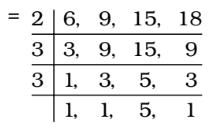Home » Aptitude » LCM and HCF » Question

#### LCM and HCF

1. The least multiple of 7, which leaves the remainder 4, when divided by any of 6, 9, 15 and 18, is
1. 76
2. 94
3. 184
4. 364
##### Correct Option: D

LCM of 6, 9, 15 and 18∴ LCM = 2 × 3 × 3 × 5 = 90
∴ Required number = 90k + 4, which must be a multiple of 7 for some value of k.
For k = 4,
Required Number = 90 × 4 + 4 = 364, which is exactly divisible by 7.